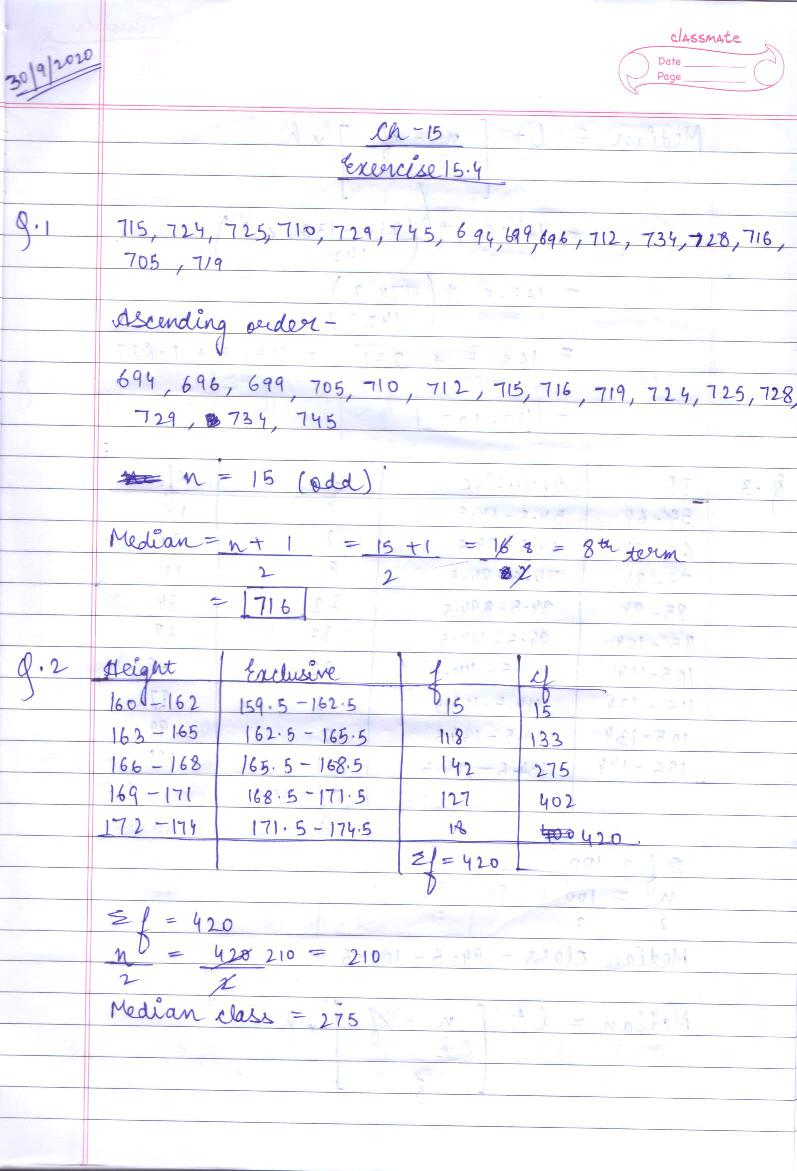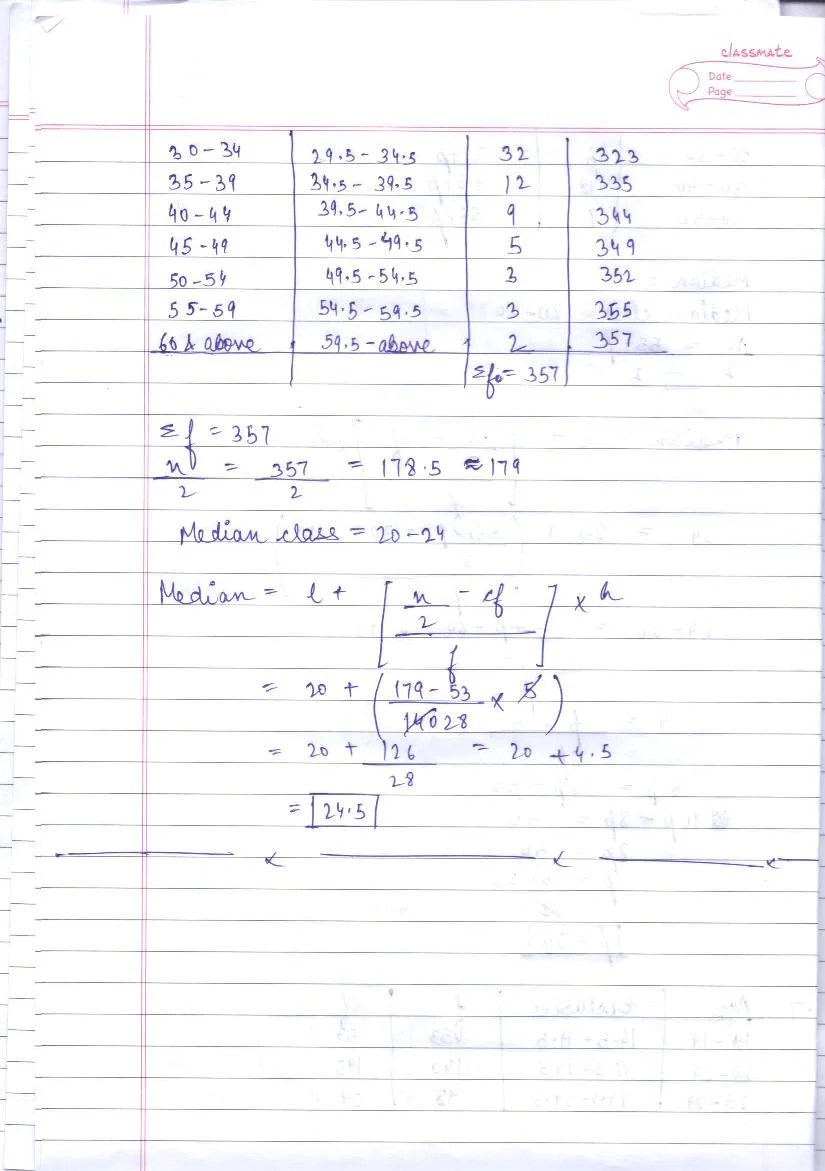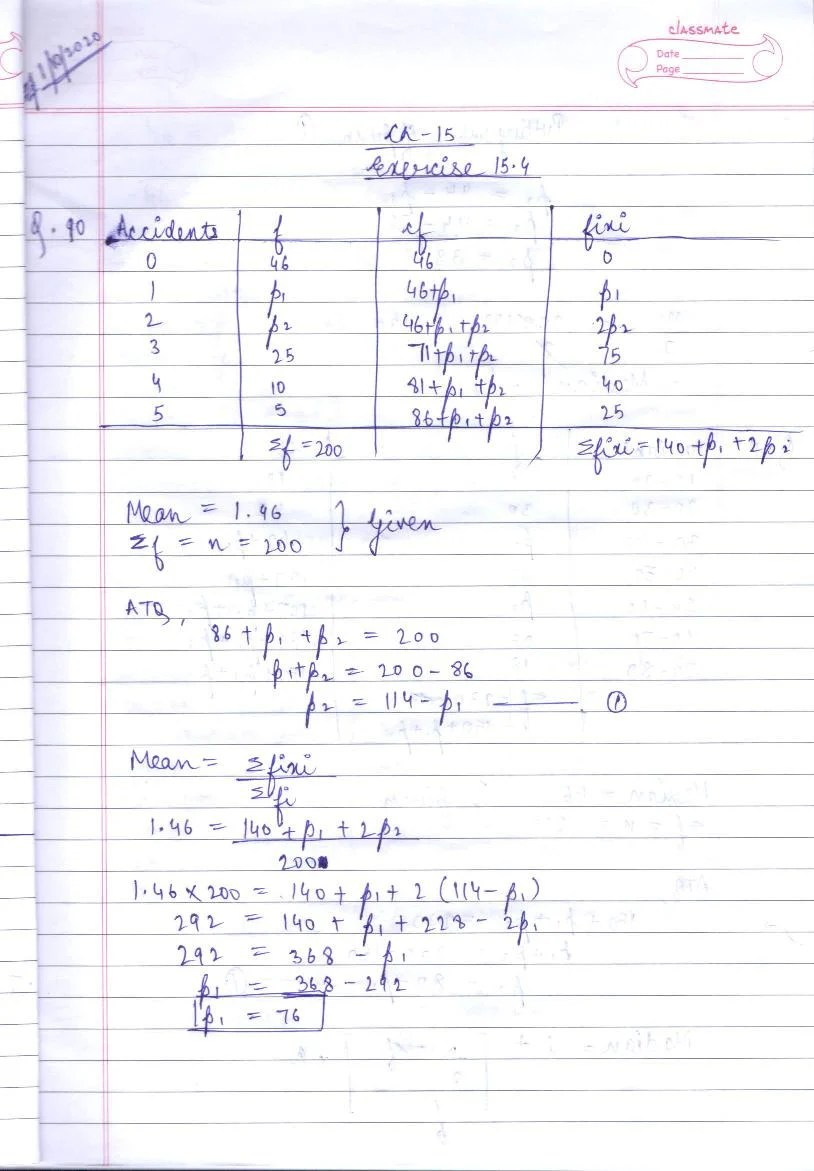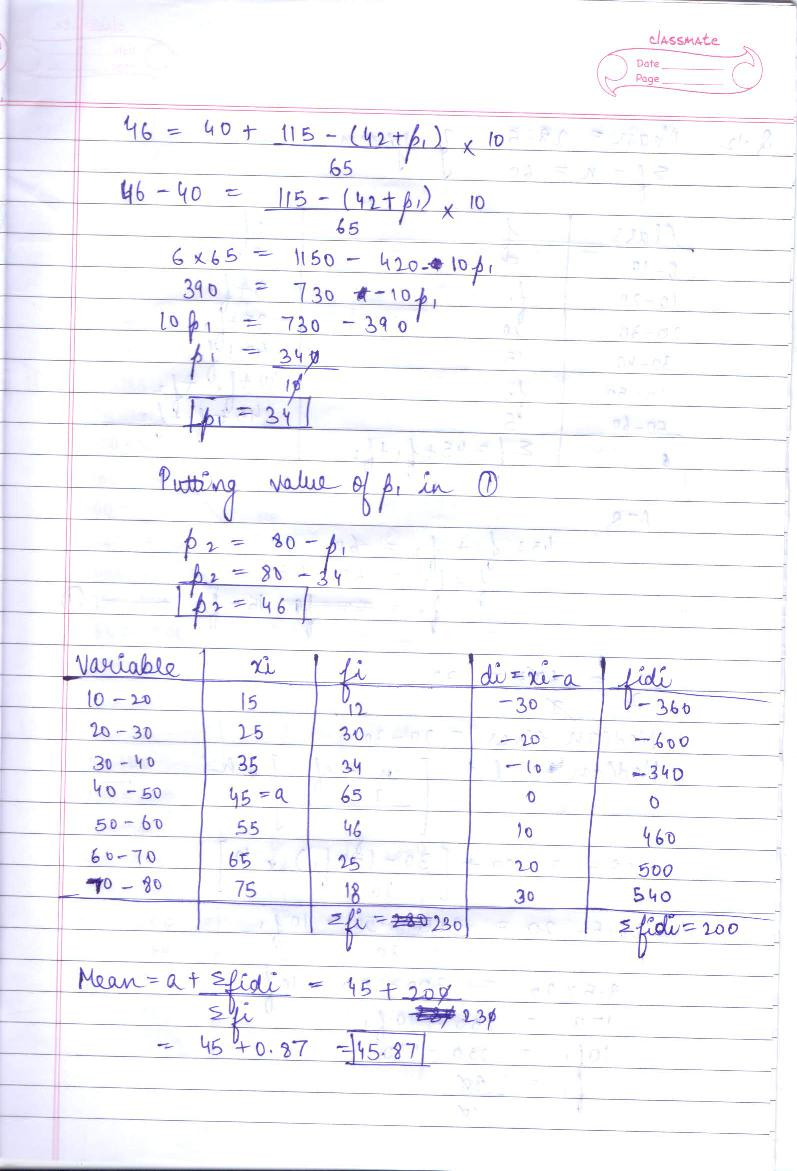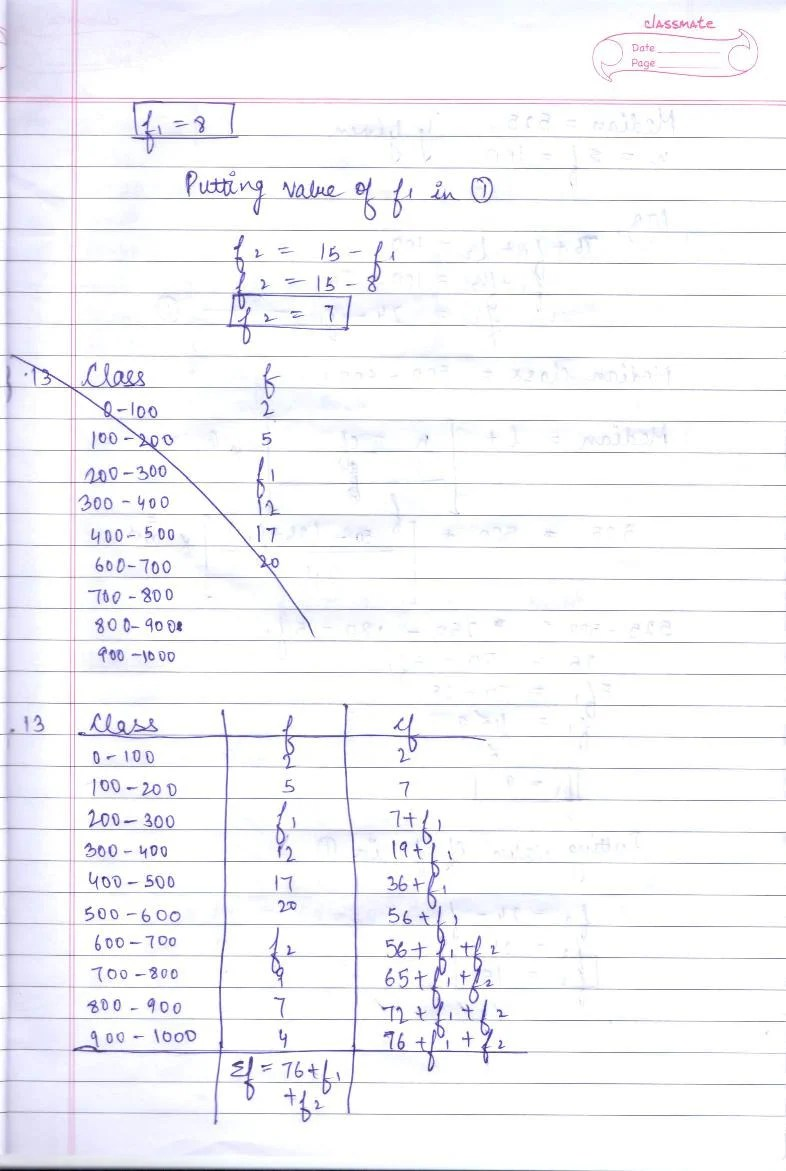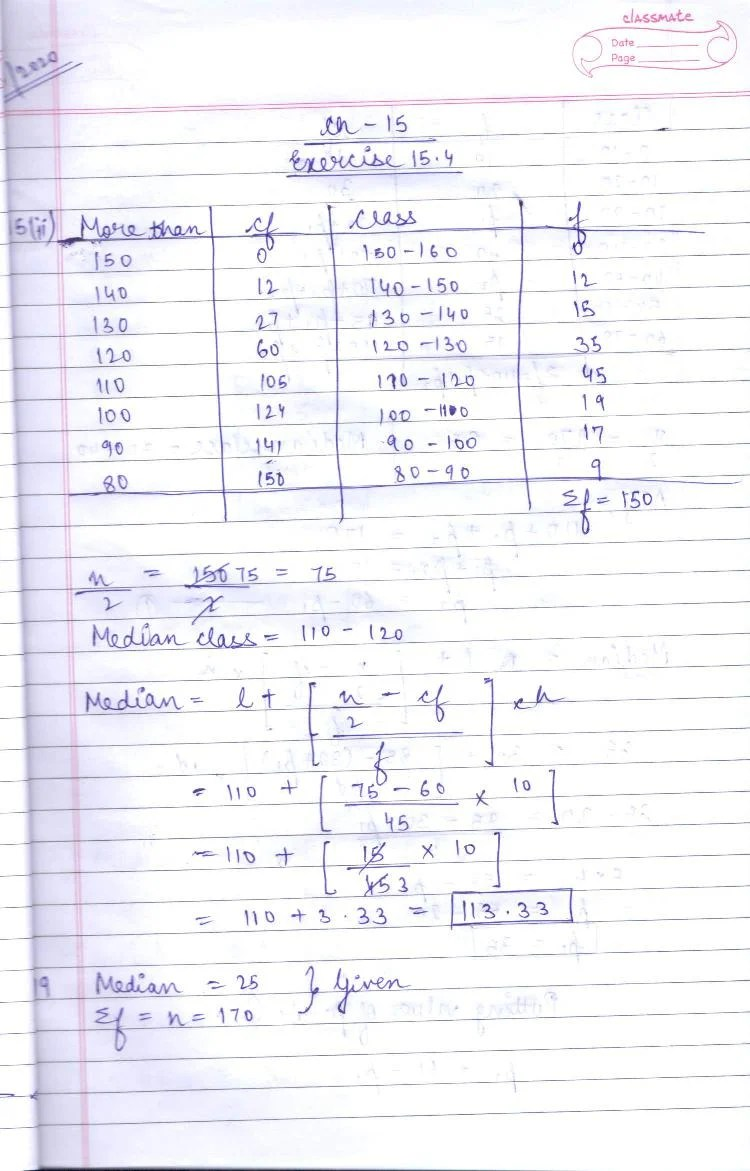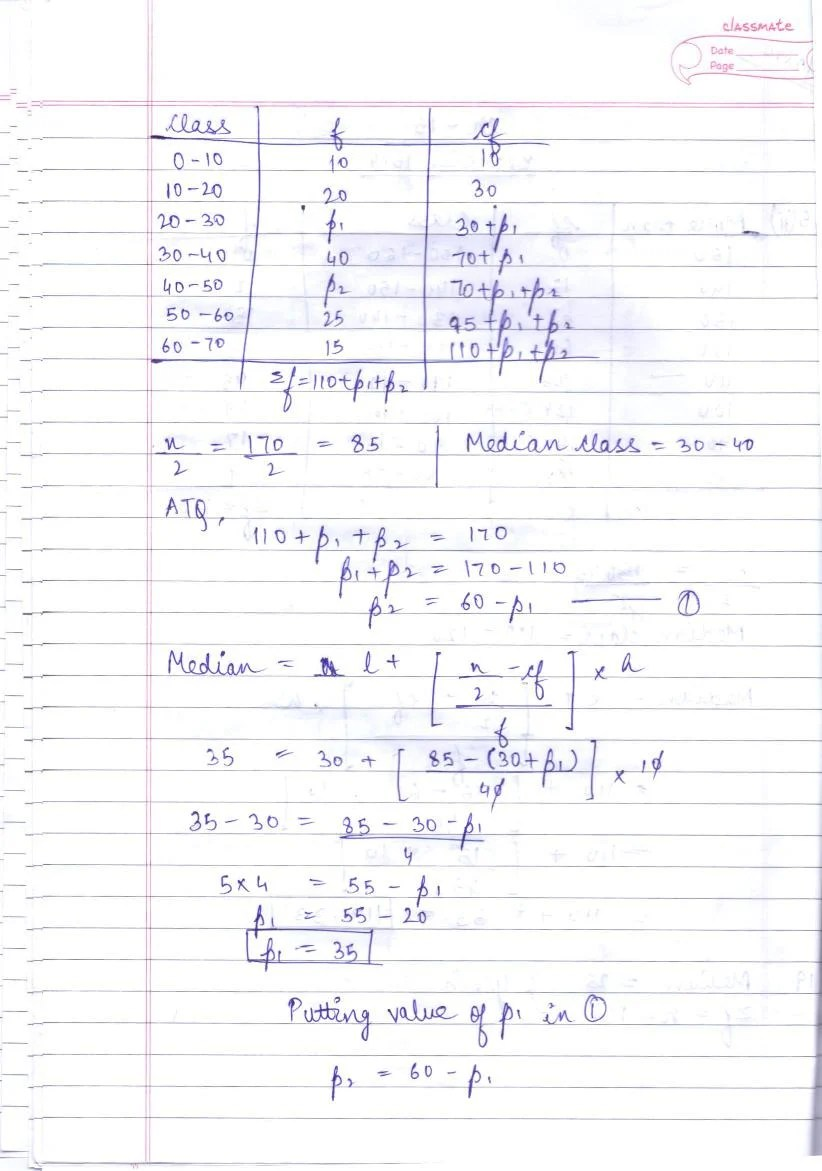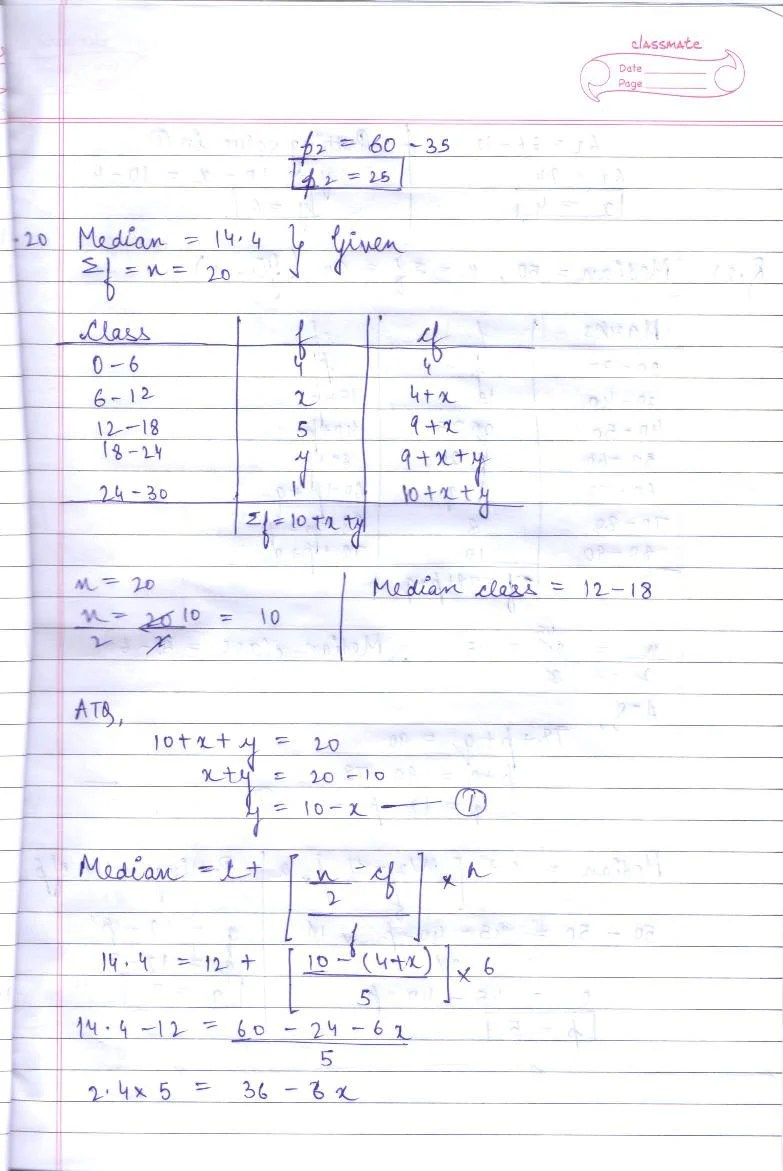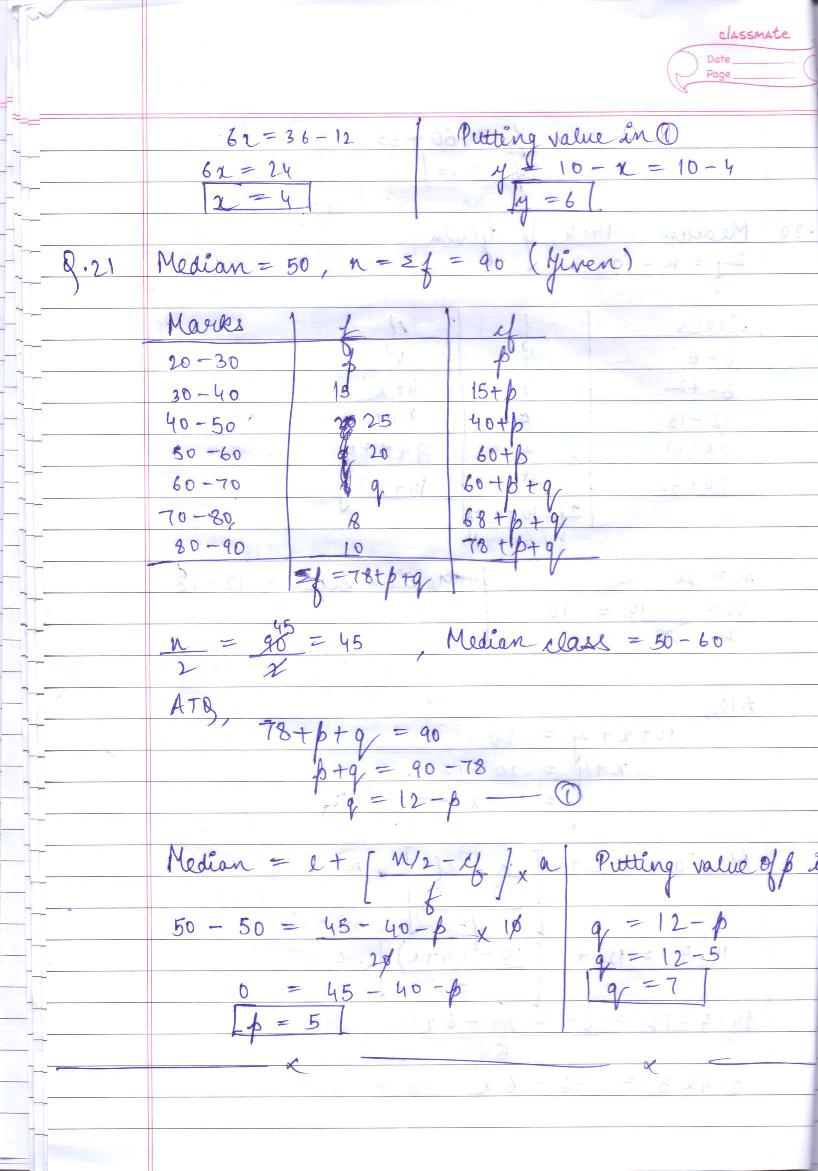# RD Sharma Solutions Class 10 Chapter 15 Statistics Exercise 15.4

Here you can get free RD Sharma Solutions for Class 10 Maths Chapter 15 Statistics Exercise 15.4. All RD Sharma Book Solutions are given here exercise wise for the chapter Statistics. RD Sharma Solutions are helpful in the preparation of several school level, graduate and undergraduate level competitive exams. Practicing questions from RD Sharma Mathematics Solutions for Class 10 Chapter 15 Statistics is proven to enhance your math skills.

 Class: Class 10th Chapter: Chapter 15 Exercise: Exercise 15.4 Name: Statistics

## RD Sharma Solutions Class 10 Chapter 15 Statistics Exercise 15.4

RD Sharma Class 10 Solutions Chapter 15 for Statistics Exercise 15.4 are given below.

RD Sharma Solutions Class 10 Chapter 15 Statistics Exercise 15.4